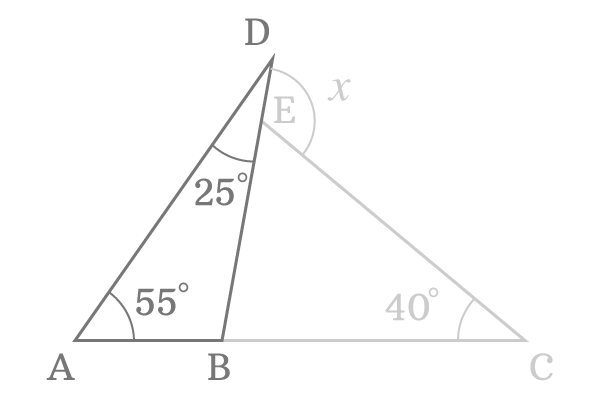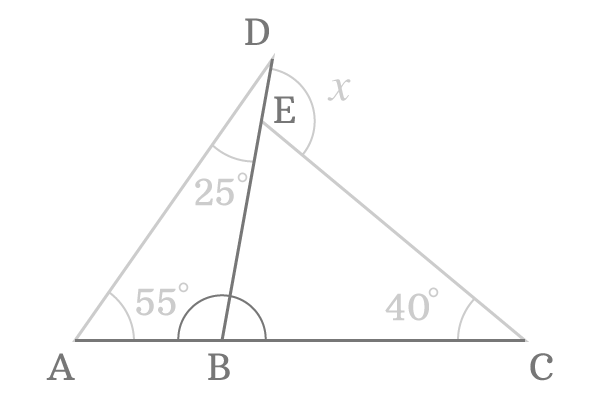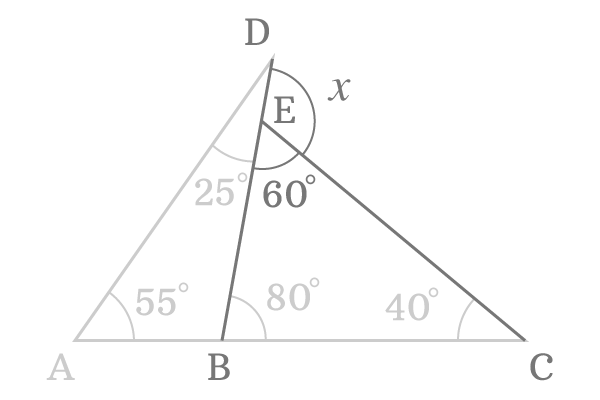# Find the common external angle of two triangles, if interior angles of one triangle are 25° and 55° and of other triangle is 40°

$\Delta ABD$ and $\Delta CBE$ are two triangles. The triangle $ABD$ has two known angles and they are $25^\circ$ and $55^\circ$ but third angle is unknown.The triangle $BCE$ has one known angle but the remaining two angles are unknown.

The two triangles has a common side $\overline{BE}$ and also have an external angle commonly and it is denoted by $x$.

In this problem, it is required to find the value of $x$ by studying the geometrical relations between angles of these two triangles.

###### Step: 1

In $\Delta ABD$, two of three angles are known and they are $25^\circ$ and $55^\circ$ but third angle is unknown. However, it can be found by applying the sum of three angles of a triangle rule.$\angle DAB + \angle ABD + \angle BDA$ $=$ $180^\circ$

The angle $DAB$ is $55^\circ$ and the angle $BDA$ is $25^\circ$. Substitute them in the equation to obtain the angle $ABD$.

$\implies 55^\circ + \angle ABD + 25^\circ$ $=$ $180^\circ$

$\implies \angle ABD + 80^\circ$ $=$ $180^\circ$

$\implies \angle ABD$ $=$ $180^\circ -80^\circ$

$\therefore \,\,\,\,\,\, \angle ABD$ $=$ $100^\circ$

It is derived that the angle $ABD$ is $100^\circ$ in the triangle $ABD$.

###### Step: 2

In triangle $BCE$, only one angle is known and it is $40^\circ$ but the two angles are unknown. If one of two angles is found, then third angle of the triangle can be easily evaluated.Now, consider the intersecting lines $\overline{AC}$ and $\overline{BD}$. Actually, $\overline{AC}$ is a straight line and its angle is $180^\circ$ geometrically. The line segment $\overline{BD}$ intersects the straight line $\overline{AC}$ at point $B$. So, it splits the angle $180^\circ$ into two sub angles and they are $\angle ABD$ and $\angle DBC$ but the angle $\angle ABD$ is $100^\circ$ as per previous step.

$\angle ABD + \angle DBC = 180^\circ$

$\implies 100^\circ + \angle DBC = 180^\circ$

$\implies \angle DBC = 180^\circ -100^\circ$

$\therefore \,\,\,\,\,\, \angle DBC = 80^\circ$

Therefore, it is derived that the angle $DBC$ is $80^\circ$ and it is the second angle of the $\Delta BCE$.

###### Step: 3

Now, the two interior angles are known for the triangle $BCE$. Apply sum of angles of the triangle rule once again to find the third angle of the triangle $BCE$.$\angle EBC + \angle BCE + \angle CEB = 180^\circ$

$\implies 80^\circ + 40^\circ + \angle CEB = 180^\circ$

$\implies 120^\circ + \angle CEB = 180^\circ$

$\implies \angle CEB = 180^\circ -120^\circ$

$\therefore \,\,\,\,\,\, \angle CEB = 60^\circ$

The third angle of the triangle $BCE$ has been evaluated in this step.

###### Step: 4$\overline{BD}$ is a straight line and its angle is $180^\circ$ but the angle is divided by the line segment $\overline{CE}$ into two parts. One of the sub angle is $\angle CEB$ and it is evaluated as $60^\circ$. So, the angle $CED$ can be evaluated geometrically.

$\angle CEB + \angle CED = \angle BED$

$\implies 60^\circ + x = 180^\circ$

$\implies x = 180^\circ -60^\circ$

$\therefore \,\,\,\,\,\, x = 120^\circ$

The value of $x$ is $120^\circ$ and it is the required solution for this geometrical problem in mathematics.

Latest Math Topics
Latest Math Problems

A best free mathematics education website for students, teachers and researchers.

###### Maths Topics

Learn each topic of the mathematics easily with understandable proofs and visual animation graphics.

###### Maths Problems

Learn how to solve the maths problems in different methods with understandable steps.

Learn solutions

###### Subscribe us

You can get the latest updates from us by following to our official page of Math Doubts in one of your favourite social media sites.